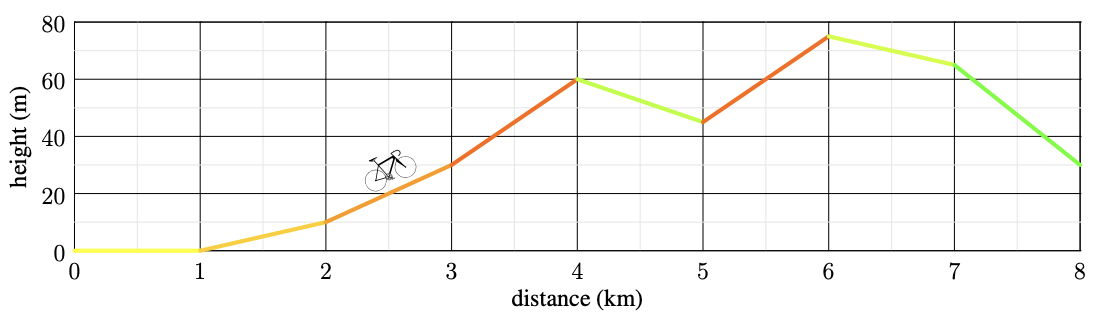시간 제한메모리 제한제출정답맞힌 사람정답 비율
6 초 512 MB101252222.680%

## 문제

The cycling classic Amstel Gold Race is held annually in the nearby Dutch province of Limburg. It features frequent short climbs giving contestants little time to recover in between. For instance the last 42 kilometres of the race has 8 steep climbs of average length roughly 1 kilometre each.

The incline grade for each climb is given in percent. An incline grade of 100% means that for every 1 horizontal metre travelled, you also travel 1 vertical metre upwards. An incline grade of 0% is perfectly flat. In general, an incline grade of p% has p/100 vertical metres for each horizontal metre. Note that the incline grade can also be negative.

You know the height of the road at integer horizontal kilometres from the start of the race, and for simplicity we model the heights as being piecewise-linear in between these points. In other words, the incline grade is assumed to be constant between 0 and 1 km, and between 1 and 2 km, and so on. For example, in Figure H.1, the height at the horizontal distance 2.5 kilometres is exactly 20 metres.Figure H.1: Illustration of Sample Input 1. The incline grades of the first 3 horizontal kilometres are in order 0%, 1% and 2%. The last kilometer has an incline grade of −3.5%.

The Amstel Gold Race cannot be compared with some hilly stages of the Tour de France or the Giro d’Italia, but you are still interested in a comparison. For each one-day race, whether it is the Amstel Gold Race or one of the stages of the Tour de France, you would like to know the length of the longest horizontal interval with at least a given incline grade. Actually, you would like to know the answer for multiple incline grades.

The incline grade of a horizontal interval is measured between the two endpoints. For example, the incline grade between kilometers 1 and 4 of the race in Figure H.1 is 2%, as 60 vertical metres are gained in 2 kilometres. This horizontal interval is also the longest one with an incline grade of at least 2%.

## 입력

The input consists of:

• One line with two integers n and k (2 ≤ n ≤ 105, 1 ≤ k ≤ 50), the horizontal length of the race in kilometres and the number of incline grades you have chosen.
• One line with n + 1 integers h0, h1, . . . , hn (0 ≤ hi ≤ 109), where hi is the height of the route in metres i horizontal kilometres from the start.
• k lines each containing a real number g (−100 ≤ g ≤ 100 and g has exactly one digit after the decimal point), an incline grade you care about.

## 출력

For each of the k given incline grades, in the same order as in the input, output the length in kilometres of the longest horizontal interval with at least this incline grade. If no suitable interval exists, output “impossible”.

Your answers should have an absolute or relative error of at most 10−6.

## 예제 입력 1

8 2
0 0 10 30 60 45 75 65 30
2.0
3.1


## 예제 출력 1

3.000000000
impossible


## 예제 입력 2

2 2
0 30 30
3.0
2.0


## 예제 출력 2

1.000000000
1.500000000


## 출처• 문제를 만든 사람: Ragnar Groot Koerkamp